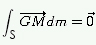NEWTON'S FIRST LAW

# LAW of INERTIA

Description: Interactive free flash animation to learn if, when the resultant force (the vector sum of all forces acting on an object) is zero, then the velocity of the object is constant.

Definition: Law of inertia: If the speed vector of the center of mass G of a solid does not vary, the sum of the forces acting on it is zero, and vice versa.

VG = cte ⇔ Σ Fext = 0

Center of mass :  We call center of mass (If the gravitational field is assumed to be uniform, here is also the center of gravity) of the material system S, the point G defined by, with M ∈ S .